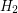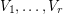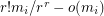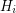Importance: Medium ✭✭
 Author(s): Kühn, Daniella Osthus, Deryk
 Subject: Graph Theory » Hypergraphs
 Keywords:
 Posted by: fhavet on: March 6th, 2013
Problem   Letandbe two-uniform hypergraph on the same vertex set. Does there always exist a partition ofintoclassessuch that for both, at leasthyperedges ofmeet each of the classes?

The bound on the number of hyperedges is what one would expect for a random partition. For graphs, the question was answered in the affirmative in [KO]. Keevash and Sudakov observed that the answer is negative if we consider many hypergraphs instead of just 2 (see [KO] for the example).

## Bibliography

*[KO] D. Kühn and D. Osthus, Maximizing several cuts simultaneously, Combinatorics, Probability and Computing 16 (2007), 277-283.

* indicates original appearance(s) of problem.